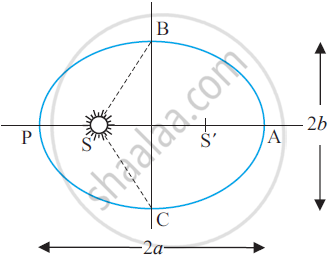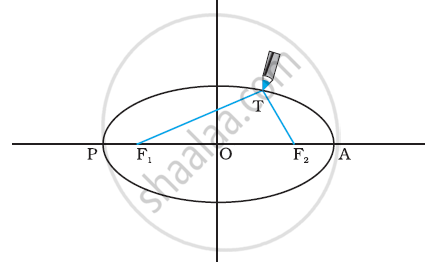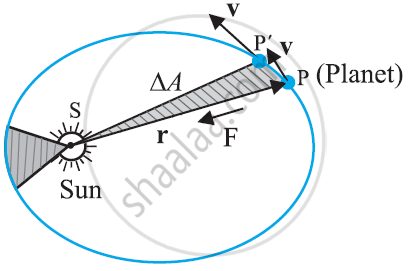# Kepler’S Laws

#### description

1. Law of orbit
2. Law of areas
3. Law of periods

### KEPLER’S LAWS

The three laws of Kepler can be stated as follows:

Kepler’s First law (Law of orbits):- All planets move in elliptical orbits with the Sun situated at one of the foci of the ellipse.Kepler’s 1st law Vs. Copernicus Model

• According to Copernicus planets move in circular motion whereas according to Kepler planets revolve in elliptical orbit around the sun.

• Copernicus model is based on one special case because circle is a special case of ellipse whereas Kepler’s laws aremore of ageneral form.

To Show ellipse is a special form of Circle• Select two points F1 and F2.

• Take a pieceof string and fix its ends at F1 and F2.

• Stretch the string taut with the help of a pencil and then draw a curve by moving the pencil keeping the string taut throughout.

• The resulting closed curve is an ellipse. For any point T on the ellipse, the sum of distances from F1 and F2 is a constant. F1,F2 are called the foci.

• Join the points F1 and F2,and extend the line to intersect the ellipse at points P and A.

• The centre point of the line PA is the centre of the ellipse O and the length PO = AO, which is also known as the semimajor axis of the ellipse.

• For a circle, the two foci merge onto one and the semi-major axis becomes the radius of the circle.

• A string has its ends fixed at F1 and F2. The tip of the pencil holdsthe string taut and is moved around and we will get an ellipse.

Kepler’s second law (law of areal velocities):-

• A planet moves round the sun in such a way that its areal velocity is constant.
• Planet moves faster when it is near to the sun and slower when it is farther from the sun.
• The areal velocity of a planet is constant.• Area covered by the planet while revolving around the sun will be equal in equal intervals of time. This means the rate of change of area with time is constant.

• Suppose position and momentum of planet is denoted by ‘r’ and ‘p’ and the time taken will be Δt.

• Delta"A"=1/2xx"r"xx"v"Delta"t" (where "v"Delta"t" is distance travelled by a planet in Delta"t" time)

(Delta"A")/(Delta"t")=1/2(rxxv)                becausev=p/m 

=1/2((rxxp))/m

= "L"/"2m"

where 'v' ia the velocity, L is the angular momentum equal to ("r"xx"p").  For a central force, which is directed along r, L is  a constant as the planet goes around. Hence, (Delta"A")/(Delta"t")is a constant according to the last equation. This is the law of areas. Gravitation is a central force and hence the law of areas follows.

Kepler’s third law (law of time period):- A planet moves round the sun in such a way that the square of its period is proportional to the cube of semi major axis of its elliptical orbit.

Statement:

Accourding to this law the square of time period of a planet is prop to the cube of the semi-major axis of its orbit.

suppose earth is revolving around the sun then the square of the time period is prop to the cube of the semi major axis.

It is known as the law of periods as it is dependent on the time period of planets.

Derivation of 3rd law: Let us assume that the path of planet is circular

let m = mass of the planet

M = mass of the sun

according to newtons law of gravitation:

"F"="GMm"/"r"^2

"F"_c = "mv"^2/r

where "F"_c= Centripetal force which helps the planet to move around the sun.

"F"="F"_c

"GMm"/"r"^2 = "mv"^2/"r"

"GM"/"r" = "v"^2         ...(1)

"v" = (2pi"r")/"T"

squaring both the sides in the above eq.

"v"^2 = (4pi^2"r"^2)/"T"^2

Substituting this value in eq (1) we get

"GM"/"r" = (4pir^2)/T^2

"T"^2 = (4pi^2"r"^3)/"GM"  Where ((4pi^2)/"GM")="constant"

"T"^2 = "r"^3`(In ellipse semi-major axis is same as radius of the circle)

If you would like to contribute notes or other learning material, please submit them using the button below.

### Shaalaa.com

introduction [01:22:25]
S
0%